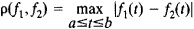# Function Space

(redirected from Function spaces)

## function space

[′fəŋk·shən ‚spās]
(mathematics)
A metric space whose elements are functions.
McGraw-Hill Dictionary of Scientific & Technical Terms, 6E, Copyright © 2003 by The McGraw-Hill Companies, Inc.
The following article is from The Great Soviet Encyclopedia (1979). It might be outdated or ideologically biased.

## Function Space

a set of functions for which there is defined a notion of distance or, more generally, of proximity between any two functions. A function space is called linear if, along with any two elements f1 and f2 it contains all their linear combinations αf1, + βf2, where α and β are real or complex numbers. An example of a linear function space is the space C(a, b) of all continuous functions on the interval [a, b] with the distance p(f1, f2) between two functions being given by the formulaFunction spaces are the most important concrete linear spaces studied in functional analysis. [28–388–1 ]

The Great Soviet Encyclopedia, 3rd Edition (1970-1979). © 2010 The Gale Group, Inc. All rights reserved.
References in periodicals archive ?
Density of Continuous Function and Trigonometric Polynomials in Banach Function Spaces. The following statement can be proved by analogy with [9, Lemma 1.3].
Narrow operators on function spaces and vector lattices.
Specific topics include Toeplitz and Hankel operators, Hilbert function spaces and Nevanlinna-Pick kernels, functional analysis, and function theory on the disk.
Four new rooms were added for private functions, and other function spaces, like the famous St.
Among the highlights are spectral, structural, and geometric properties of special types of operators on Banach spaces, emphasizing isometries, weighted composition operators, and projections on function spaces. Among specific topics are sparse hamburger moment multi-sequences, surjective isometries on absolutely continuous vector valued function spaces, extensions of isometries in generalized gyrovector spaces of the positive cones, kernels of adjoints of composition operators with rational symbols of degree two, and associating linear and nonlinear operators.
From vector spaces to function spaces; introduction to functional analysis with applications.
The results include such themes as frame theory and applications, harmonic analysis and function spaces, harmonic analysis and number theory, integral geometry and Radon transforms, and multi-resolution analysis, wavelets and applications.
He covers the basic elements of metric topology, new types of function spaces, the theory of Hilbert spaces, operators in Hilbert spaces, spectral theory, Fredholm theory, and a wide variety of other related subjects over the course of the bookAEs six chapters.
Semismooth Newton methods for variational inequalities and constrained optimization problems in function spaces.
The Baouendi- Treves approximation theory is proved for many function spaces and applied to questions in partial differential equations and complex variables.
Conference on Function Spaces (6th: 2010: Edwardsville, IL) Ed.
Topics include complex variables and potential theory (featuring integral representations in a range of analyses methods and nonlinear potential theory in metric spaces), differential equations and nonlinear analysis (mean curvature flow, bifurcation theory, a nonlinear eigenvalue problem, nonlinear elliptic equations with critical and supercritical Sobolev exponents, eigenvalue analysis of elliptical operators and the theory of nonlinear semigroups), and harmonic analysis (integral geometry and spectral analysis, Fourier analysis and geometric combinatories, eigenfunctions of the Laplacian, fractal analysis via function spaces and five reviews of harmonic analysis techniques).

Site: Follow: Share:
Open / Close